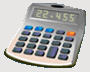DosageHelp.com Helping Nursing Students Prepare for Medication Exams By Explaining Dosage Calculations

# Unit Conversion

Mass:
• mcg → mg → g → kg    ( ÷ by 1,000 )
• mcg ← mg ← g ← kg    ( x by 1,000 )
• lb → kg    ( ÷ by 2.2 )
• lb ← kg    ( x by 2.2 )
Volume:
• mcL → mL → L → kL    ( ÷ by 1,000 )
• mcL ← mL ← L ← kL    ( x by 1,000 )
Time:
• min → hr    ( ÷ by 60 )
• min ← hr    ( x by 60 )

Example: Convert 5,000 mcg to mg.
• mcg → mg → g → kg    ( ÷ by 1,000 )
• 5,000 mcg ÷ 1,000 = 5 mg

Example: Convert 44 lb to kg.
• lb → kg    ( ÷ by 2.2 )
• 44 lb ÷ 2.2 = 20 kg

Example: Convert 0.003 L to mcL.
• mcL ← mL ← L ← kL    ( x by 1,000 )
• 0.003 L x 1,000 = 3 mL
• 3 mL x 1,000 = 3,000 mcL

Example: Convert 5 hours to minutes.
• min ← hr    ( x by 60 )
• 5 hr x 60 = 300 min

Home Dosage Question Steps Unit Conversion Mass for Mass Mass/Liquid For Liquid IV Terms & Abbreviations Amount in IV Fluid Volume/Time - IV mL Rate Volume/Time - IV Drop Rate Fluid Maintenance Dosage By Weight Mass/Time - IV mL Rate Practice Questions Question of the Day Tell a Friend Print a Flyer